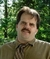## Write Python program for sum of seriesTags:
Python
How can I write a Python program to find the sum of the this series: - X - X**2/2! + X**3/3! - X**4/4! + X**5/5! - X**6/6! Thanks!

Thanks. We'll let you know when a new response is added.

## Discuss This Question: 12 Replies

Thanks. We'll let you know when a new response is added.
•Truly this is homework and you have to try it by reading your study books. Or you can navigate python.org for learning more.
report
• Well My Source Code Has Some Syntax Error Except For Indentations. That's why I had asked for a proper source code for the given question.
report
• X = int(raw_input("Enter the value for x:"))
j = int(raw_input("Enter The value for j:"))
Sum = 0
Fact = 1
Sign = 1
for i in range(1,x+1):
for i in range(1,j+1):
Fact = Fact*1
Sum += (Sign*(X**i)/Fact*j
report
• There is syntax error in the following source code(Except for indentations). Even if you correct them, there still persists some syntax errors. Please help me correct them.
report
•You should post the exact error messages you are getting. That is always a must-do thing when asking for help on technical problems.
report
• X = int(raw_input("Enter the value for x:"))
j = int(raw_input("Enter The value for j:"))
Sum = 0
Fact = 1
Sign = 1
for i in range(1,x+1):
for i in range(1,j+1):
Fact = Fact*1
Sum += (Sign*(X**i)/Fact*j
Here, a red mark comes saying there is a syntax error
report
•Where exactly is "here"?
report
• Okay never mind, can you please write a proper source code for this program and post it :)
report
•The problem is that it is your homework, and we should not do it for you.

It is easy to ask for help the right way, when it comes to homework questions:

Post your code, as you did, then tell us the exact problem you are having, and if you are getting errors, post the exact and complete error message you are getting, and if possible, the line of code that is causing the problem or being reported as incorrect.

Then we can try to help you without doing the whole work for you.

Does it make sense?
report
•Your last line is missing the close parenthesis

Sum += (Sign*(X**i)/Fact*j)
report
• Why don't you refer the tutorials available online related to this and I am sure you will find the solution.
report
• # Sum of the this series:- X - X**2/2! + X**3/3! - X**4/4! + X**5/5! - X**6/6!
x = int(input("Enter the value for x:"))
#j = int(input("Enter The value for j:"))
Sum = 0
Fact = 1
Sign = 1
for i in range(1,x+1):
#    for j in range(1,j+1):
Fact = Fact*1
Sum += (Sign*(x**i)/Fact*i)
print(Sum)

report